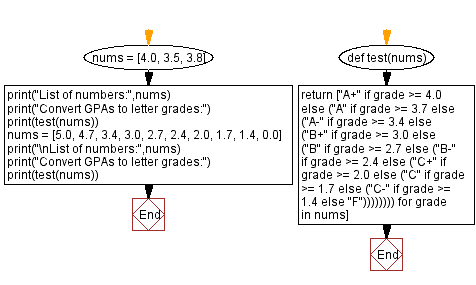﻿ Python: Convert GPAs to letter grades - w3resource# Python: Convert GPAs to letter grades

## Python Programming Puzzles: Exercise-77 with Solution

Write a Python program to convert GPAs to letter grades according to the following table:

4.0: A+
3.7: A
3.4: A-
3.0: B+
2.7: B
2.4: B-
2.0: C+
1.7: C
1.4: C-
below:F
```Input:
[4.0, 3.5, 3.8]
Output:
['A+', 'A-', 'A']
Input:
[5.0, 4.7, 3.4, 3.0, 2.7, 2.4, 2.0, 1.7, 1.4, 0.0]
Output:
['A+', 'A+', 'A-', 'B+', 'B', 'B-', 'C+', 'C', 'C-', 'F']
```

Sample Solution:

Python Code:

``````#License: https://bit.ly/3oLErEI

def test(nums):
return ["A+" if grade >= 4.0
else ("A" if grade >= 3.7
else ("A-" if grade >= 3.4
else ("B+" if grade >= 3.0
else ("B" if grade >= 2.7
else ("B-" if grade >= 2.4
else ("C+" if grade >= 2.0
else ("C" if grade >= 1.7
else ("C-" if grade >= 1.4
else "F"))))))))

nums = [4.0, 3.5, 3.8]
print("List of numbers:",nums)
print(test(nums))
nums = [5.0, 4.7, 3.4, 3.0, 2.7, 2.4, 2.0, 1.7, 1.4, 0.0]
print("\nList of numbers:",nums)
print(test(nums))
``````

Sample Output:

```List of numbers: [4.0, 3.5, 3.8]
['A+', 'A-', 'A']

List of numbers: [5.0, 4.7, 3.4, 3.0, 2.7, 2.4, 2.0, 1.7, 1.4, 0.0]
['A+', 'A+', 'A-', 'B+', 'B', 'B-', 'C+', 'C', 'C-', 'F']
```

Flowchart:## Visualize Python code execution:

The following tool visualize what the computer is doing step-by-step as it executes the said program:

Python Code Editor :

Have another way to solve this solution? Contribute your code (and comments) through Disqus.

What is the difficulty level of this exercise?

Test your Programming skills with w3resource's quiz.

﻿

## Python: Tips of the Day

Clamps num within the inclusive range specified by the boundary values x and y:

Example:

```def tips_clamp_num(num,x,y):
return max(min(num, max(x, y)), min(x, y))
print(tips_clamp_num(2, 4, 6))
print(tips_clamp_num(1, -1, -6))
```

Output:

```4
-1
```